## 10.4.3 Q-Learning: Computing an Optimal Plan

This section moves from evaluating a plan to computing an optimal plan in the simulation-based framework. The most important idea is the computation of Q-factors,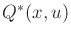. This is an extension of the optimal cost-to-go,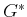, that records optimal costs for each possible combination of a state,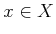, and action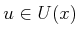. The interpretation ofis the expected cost received by starting from state, applying, and then following the optimal plan from the resulting next state,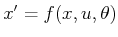. Ifhappens to be the same action as would be selected by the optimal plan,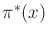, then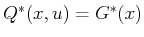. Thus, the Q-value can be thought of as the cost of making an arbitrary choice in the first stage and then exhibiting optimal decision making afterward.

Subsections

Steven M LaValle 2020-08-14